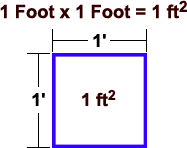Triangle Square Footage CalculatorWhat is a Square Foot?

A "square foot" is a unit of area measurement equal to a square measuring one foot on each side. 1 Square Foot = 0.0929 square meters. Abbrev.: ft2, sq.ft, SqFt.